Vectors and conics

This free course is available to start right now. Review the full course description and key learning outcomes and create an account and enrol if you want a free statement of participation.

Free course

2.4 Components and the arithmetic of vectors

We introduce now a different method of representing vectors, which will make the manipulation of vectors much easier. Thus we shall avoid having to solve problems involving vectors by drawing the vectors and making measurements, which is very time-consuming and never very accurate.

We can think of a vector as a translation, that is, as representing a movement by a certain amount in a given direction. Then we can use the Cartesian axes in the plane or in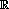3 to describe the translation.

For example, consider the vector v, in2, shown below. In view of the Triangle Law for adding vectors, we can think of v as the sum of two vectors, one of magnitude 3 units in the direction of the positive x-axis and one of magnitude 4 units in the direction of the positive y-axis. (In this sense, we can associate the ordered pair of real numbers (3, 4) with the vector v.)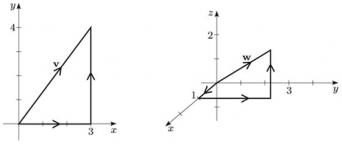Similarly, in3, the vector w shown above can be considered as the sum of three vectors, one of magnitude 1 in the direction of the positive x-axis, one of magnitude 3 in the direction of the positive y-axis, and one of magnitude 2 in the direction of the positive z-axis. So we can associate the ordered triple of real numbers (1, 3, 2) with the vector w.

Thus we can express a vector in2 in terms of multiples of unit vectors (vectors of magnitude 1 unit) in the directions of the x- and y-axes, and a vector in3 in terms of multiples of unit vectors in the directions of the x-, y- and z-axes. This expression of a vector as a sum of vectors in standard directions is called decomposition of the vector into components. This representation of a vector in terms of components will be useful in Section 3.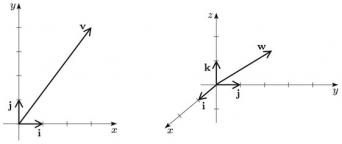Definitions

In2, the vectors i and j are unit vectors in the positive directions of the x- and y-axes, respectively. Any vector p in2 can be expressed as a sum of the form

p = a1i + a2j, for some real numbers a1, a2;

often we write p = (a1, a2), for brevity. The numbers a1 and a2 are the components of p in the x- and y-directions, respectively.

In3, the vectors i, j and k are unit vectors in the positive directions of the x-, y- and z-axes, respectively. Any vector p in3 can be expressed as a sum of the form

p = a1i + a2j + a3k, for some real numbers a1, a2, a3;

often we write p = (a1, a2, a3), for brevity. The numbers a1, a2 and a3 are the components of p in the x-, y- and z-directions, respectively.

Using these definitions, we can express the above vector v as 3i + 4j, or simply as (3, 4).

Also using these definitions, we can express the above vector w as i + 3j + 2k, or simply as (1, 3, 2).

It is important to remember that the above expression of a vector as an ordered pair or an ordered triple of real numbers simply describes the components of the corresponding translation; it does not mean that we are identifying the vector with the point whose Cartesian coordinates are that pair or triple.

Example 22

Sketch the following vectors in2 on a single diagram:

2i − 3j, −3i + 4j, −2i − 2j.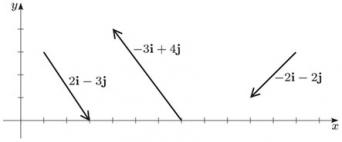In the above discussion we expressed vectors in2 and in3 in terms of components relative to unit vectors that are mutually perpendicular. In general, however, we do not need to restrict ourselves in this way. In fact, we can express a vector in2 in terms of any two vectors that are not parallel (that is, whose directions are not the same or exactly opposite), and a vector in3 in terms of any three non-coplanar vectors. (A set of vectors in3 is coplanar if there is some plane in3 that contains all the vectors of the set).

Now that we can describe vectors in terms of their components, we return to the operations on vectors which we described geometrically in Sections 2.1 through 2.3, in order to describe the same operations in terms of components.

The following observations follow immediately from the definition of components.

Definitions

Two vectors, both in2 or both in3, are equal if and only if their corresponding components are equal.

The zero vector in2 is 0 = 0i + 0j = (0, 0), and the zero vector in3 is 0 = 0i + 0j + 0k = (0, 0, 0).

We have described addition of vectors already. To add two vectors p and q using the Triangle Law, we first draw the vector p; then, starting from the finishing point of p, we draw the vector q. The sum p + q is the vector from the starting point of p to the finishing point of q.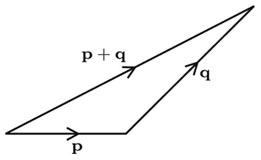Recall that we can think of a vector as a translation.

In order to see how addition can be described in terms of components, we look at the sum of two vectors in the plane. Let p and q be the vectors pa1i + a2j = (a1,a2), q = b1i + b2j = (b1,b2).

Then a translation p followed by a translation q can be described in terms of the following four successive translations:

• move a1 units in the direction of the positive x-axis (this translation is vector a1i);

• then a2 units in the direction of the positive y-axis (this is a2j);

• then b1 units in the direction of the positive x-axis (this is b1i);

• then b2 units in the direction of the positive y-axis (this is b2j).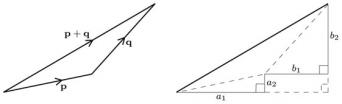Since the order in which we perform translations does not matter, the net effect is a translation of a1 + b1 units in the positive direction of the x-axis, followed by a translation of a2 + b2 units in the positive direction of the y-axis. In other words, the sum p + q is the vector (a1, a2) + (b1, b2) = (a1 + b1, a2 + b2).

There is a corresponding formula in3.

To add vectors in2 or in3 given in component form, add their corresponding components: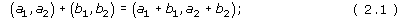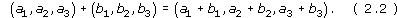Note: There are analogous formulas for vectors expressed in terms of the unit vectors i and j, or i, j and k.

For example, the sum of the vectors (1, −3) and (4, 2) in2 is the vector (1 + 4, −3 + 2) = (5, −1), and the sum of the vectors (2, −1, −3) and (−2, 3, 2) in3 is the vector (2 − 2, −1 + 3, −3 + 2) = (0, 2, −1).

In equations (2.1) and (2.2) above, the symbol + is used with two different meanings. On the left-hand side of each equation, + denotes addition of vectors, and on the right-hand side, + denotes addition of real numbers. It is sensible to use the same symbol, as addition of vectors obeys the same rules as addition of real numbers.

Example 23

Determine the following sums of vectors in2: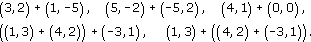(3,2) + (1,−5) = (4,−3),

(5,−2) + (−5,2) = (0,0),

(4,1) + (0,0) = (4,1),

((1,3) + (4,2)) + (−3,1) = (5,5) + (−3,1) = (2,6),

(1,3) + ((4,2) + (−3,1)) = (1,3) + (1,3) = (2,6).

You saw in Exercise 23 (above) that the sum of (5, −2) and (−5, 2) is the zero vector. In general, in2 the sum of the vectors p = (a1, a2) and (−a1, −a2) is the zero vector, so that in terms of its components, the vector −p is (−a1, −a2). Similarly, in terms of components, the negative of the vector p = (a1, a2, a3) in3 is −p = (−a1, −a2, −a3). For example, if p = (1, −3), then −p = (−1, 3); and if p = (2, −1, 3), then −p = (−2, 1, −3).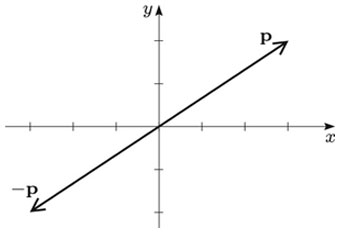We can then express the difference pq of two vectors p and q in terms of components since, as we saw in Section 2.3, pq is defined to be p + (−q).

For example, if p = (4, 1) and q = (2, −1), then −q = (−2, 1) and

pq = (4 − 2,1 + 1) = (2, 2).

Similarly, if p = i + 2j − 3k and q = 2ij + k, then −q = −2i + jk and

pq = −i + 3j − 4k.

Negative of a vector

To find the negative of a vector in2 or in3 given in component form, take the negatives of its components: −(a1, a2) = (−a1, −a2)

and −(a1, a2, a3) = (−a1, −a2, −a3).

Subtraction of vectors

To subtract a vector in2 or in3 given in component form, subtract its corresponding components: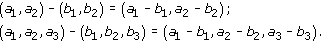For the above formulas, there are analogous formulas for vectors expressed in terms of the unit vectors i and j, or i, j and k.

Example 24

For each of the following pairs of vectors p and q, write down −p, −q and pq.

• (a) p = (3, −1) and q = (−1, −2).

• (b) p = −i − 2j and q = 2ij.

• (c) p = −i + 2k and q = i − 2jk.

• (a) Here p = (3, −1) and q = (−1, −2), so

p = (−3,1),

q = (1,2),

pq = (3 + 1,−1 + 2) = (4,1).

• (b) Here p = −i − 2j and q = 2ij, so

p =i+ 2j,

q = −2i +j,

pq = (−1 − 2)i + (−2 + 1)j = −3i −j.

• (c) Here p = −i + 2k and q = i − 2jk, so

p = i − 2k,

q = −i + 2j + k,

pq = −2i + 2j + 3k.

The other operation that we described geometrically earlier was multiplication by a scalar. For example, the vector 2p has magnitude twice that of p and has the same direction; so, in terms of components, if p = (a1, a2), then 2p = (2a1, 2a2).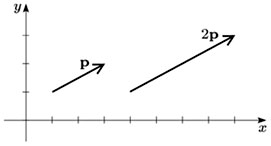In general, if a vector in2 or in3 is multiplied by a scalar k, then each of its components is multiplied by k. Recall that the product kp is in the same direction as p if k is positive, and in the opposite direction if k is negative.

Multiplication by a scalar

To multiply a vector given in component form in2 or in3 by a real number k, multiply each component in turn by k: k(a1, a2) = (ka1, ka2)

and k(a1, a2, a3) = (ka1, ka2, ka3).

Note: There are analogous formulas for vectors expressed in terms of the unit vectors i and j, or i, j and k.

For example, if p = (2, −1), then 2p = (4, −2) and −3p = (−6, 3).

Example 25

For each of the following pairs of vectors p and q, determine 2p, 3q, 2p + 3q and 2p − 3q.

• (a) p = (3, −1) and q = (−1, −2).

• (b) p = −i + 2k and q = i − 2jk.

• (a) Since p = (3, −1) and q = (−1, −2),

2p = (6,−2),

3q = (−3,−6),

2p + 3q = (3,−8),

2p − 3q = (9,4).

• (b) Since p = −i + 2k and q = i − 2jk,

2p = −2i + 4k,

3q = 3i − 6j − 3k,

2p + 3q = i − 6j + k,

2p − 3q = −5i + 6j + 7k.

The set of ordered pairs (triples) of real numbers, together with the operations of addition of ordered pairs (triples) and multiplication of an ordered pair (triple) by a scalar, is an example of the algebraic structure known as a vector space.

Definitions

The vector space2 is the set of ordered pairs of real numbers with the operations of addition and multiplication by a scalar defined as follows: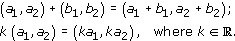Similarly, the vector space3 is the set of ordered triples of real numbers with analogous operations of addition and multiplication by a scalar.

M208_1